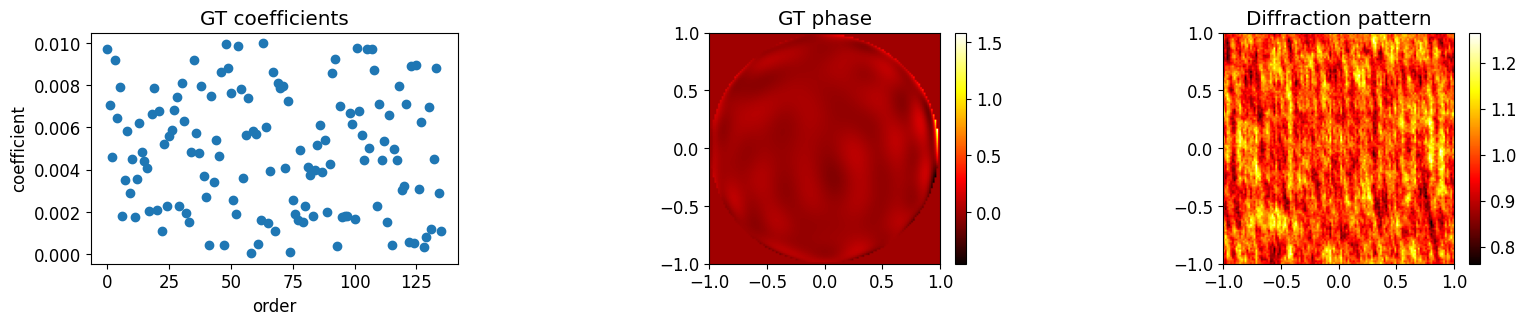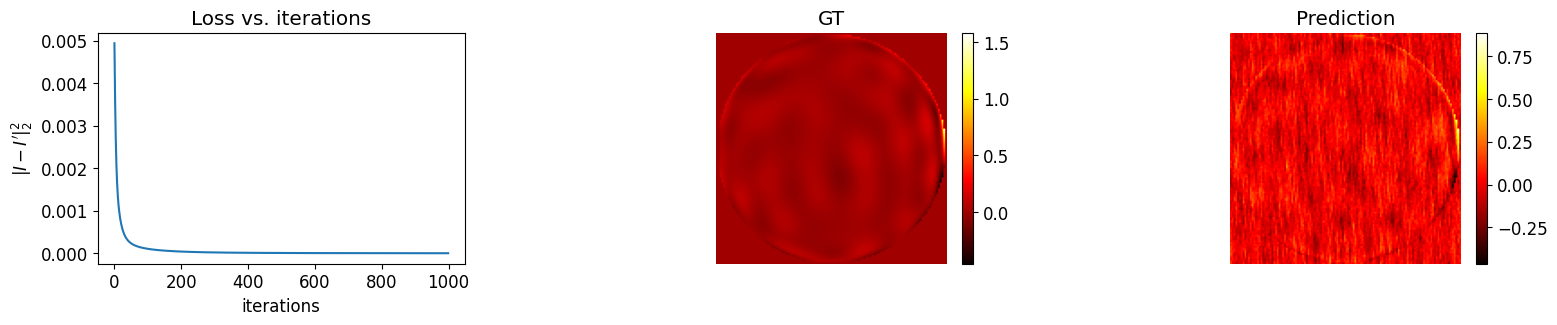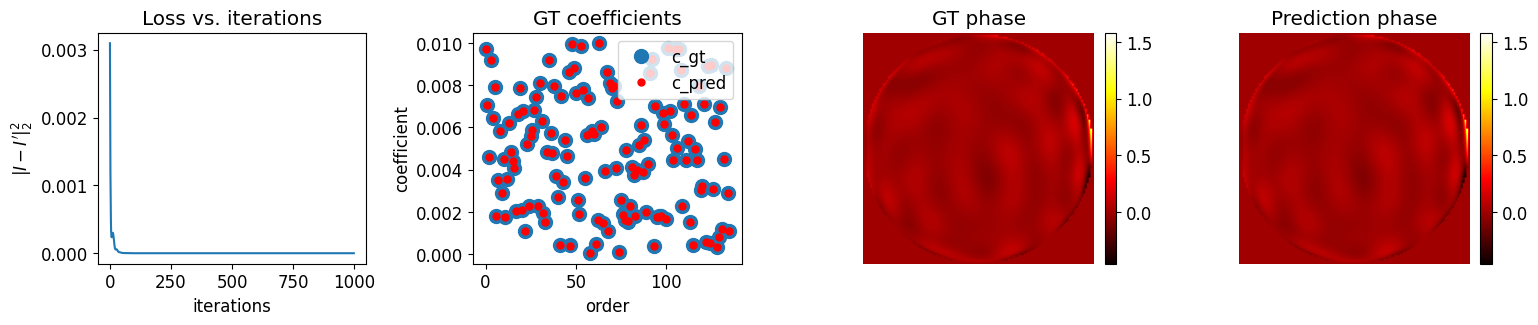## Differentiable Imaging ($\partial \mathcal{I}$): a new tool for computational optical imaging

Computational imaging has made significant advancements in recent years, but faces limitations due to the restrictions imposed by traditional computational techniques. Differentiable programming offers a promising solution by combining the strengths of classical optimization and deep learning. By integrating physics into the modeling process, differentiable imaging ($\partial \mathcal{I}$)  - which employs differentiable programming in computational imaging - has the potential to overcome challenges posed by sparse, incomplete, and noisy data. This could be a key factor in advancing the field of computational imaging and its various applications.

Modeling the physics accurately is of great importance in differentiable imaging. Since many computational imaging techniques rely on ray tracing and diffraction , we have developed both a ray-tracing differentiable framework  and a diffraction-based differentiable framework . These frameworks have applications in self-calibration , end-to-end lens design , metrology , holography ($\partial \mathcal{H}$) , and more. The differentiable ray-tracing framework has proven to be highly efficient and enables easy incorporation of neural networks, thereby promoting lens design .

Please refer to the paper to find how this differentiable imaging ($\partial \mathcal{I}$) enables more efficient and effective imaging, and our insights on its potential impact in the computational imaging field. Below is the supplementary code  of the paper.

## Example 1: How to get the derivatives in PyTorch

This is the code of the example in Fig. 3. We calculate $\frac{\partial y}{\partial x_1}$ and $\frac{\partial y}{\partial x_2}$ at $(x_1, x_2)=(2,1)$ for function of $y = \sin(x_1) + x_1 \times x_2$

import torch

x1 = torch.tensor([2.])
x2 = torch.tensor([1.])

vm = x1
v0 = x2
v1 = torch.sin(vm)
v2 = vm * v0
v3 = v1 + v2
y = v3

y.backward()



The output is

partial v3: 1.0
partial v2: 1.0
partial v1: 1.0
partial v0: 2.0
partial vm: 0.5838531255722046


## Example 2: Optimize a phase from a diffraction pattern

Suppose a diffraction pattern $y=\mathcal{P} \lbrace \exp( j \phi ) \rbrace$, we would like to get $\phi$ from $y$. We conduct two approaches:

1. Optimize $\phi$ from $y$
2. Optimize Zernike coefficients $c$ from $y$

### Generate a diffraction image from an phase abberation

import torch
from matplotlib import pyplot as plt
import torch.optim as optim
from torch import nn

from function.diffraction import *
from function.util import *

from function.zernike import RZern

torch.manual_seed(0)

############################### Generate Zernike phase ################################
cart = RZern(15)
Ny, Nx = 128, 128
xx, yy = np.meshgrid(np.linspace(-1.0, 1.0, Nx), np.linspace(-1.0, 1.0, Ny))
cart.make_cart_grid(xx, yy)

c_gt = 0.01 * torch.rand(cart.nk, dtype=torch.double)    # ground truth Zernike coefficients
phi_gt_2d = cart.eval_grid(c_gt, matrix=True).transpose(0, 1).reshape((Nx, Ny))   # ground truth phase

######################### calculate diffraction intensity from phase ########################
params = PropParam()
params.wavelen = torch.tensor([500e-9])
params.pps = torch.tensor([5e-6])
params.z = torch.nn.Parameter(torch.tensor([100e-3], dtype=torch.float32))
params.Nx, params.Ny = Nx, Ny
params = prop_kernel(params)

def forward_model_phase(phi):
field = torch.exp(1j*phi)
diffraction_pattern = forward_wave_prop(field, params).abs()**2

return diffraction_pattern

diffraction_pattern = forward_model_phase(phi_gt_2d)

# display images
plt.rcParams.update({'font.size': 12})
plt.figure(figsize=(18, 3))

plt.subplot(131)
plt.plot(c_gt, 'o')
plt.title('GT coefficients')
plt.xlabel('order')
plt.ylabel('coefficient')

plt.subplot(132)
plt.imshow(phi_gt_2d.detach(), origin='lower', extent=(-1, 1, -1, 1), cmap='hot')
plt.title('GT phase')

plt.subplot(133)
plt.imshow(diffraction_pattern.detach(), origin='lower', extent=(-1, 1, -1, 1), cmap='hot')
plt.title('Diffraction pattern')

plt.show()


Outputs:### Optimize phase directly

phi_pred = nn.Parameter(0.05*torch.rand([Nx, Ny], dtype=torch.double, requires_grad=True))

loss_hist = []
lr = 0.005
for iter in range(1000):
optimizer.zero_grad()     # Essential for update the derivatives

I_prime = forward_model_phase(phi_pred)
loss = torch.mean((I_prime - diffraction_pattern)**2)

loss_hist.append(loss.detach())
loss.backward(retain_graph=True)     # Calculate the derivatives

optimizer.step()

if iter % 100 == 0:
print("iter = {}: loss = {}, d_phi={}".format(iter, loss.data.numpy(), phi_pred.grad.mean().numpy()))

#########################################################################################
plt.rcParams.update({'font.size': 12})
plt.figure(figsize=(18, 3))

plt.subplot(131)
plt.plot(loss_hist)
plt.title('Loss vs. iterations')
plt.xlabel('iterations')
plt.ylabel(r'$|I- I^\prime|_2^2$')

plt.subplot(132)
plt.imshow(phi_gt_2d.detach(), origin='lower', extent=(-1, 1, -1, 1), cmap='hot')
plt.axis('off')
plt.title('GT')

plt.subplot(133)
plt.imshow(phi_pred.detach(), origin='lower', extent=(-1, 1, -1, 1), cmap='hot')
plt.axis('off')
plt.title('Prediction')
plt.show()


Outputs:

iter = 0: loss = 0.004936623673881058, d_phi=4.301339185275744e-23
iter = 100: loss = 0.00010576813928532341, d_phi=-1.809457589959748e-25
iter = 200: loss = 4.132147593978769e-05, d_phi=-5.363749284523539e-25
iter = 300: loss = 2.0701707083954387e-05, d_phi=-1.9516292577422997e-24
iter = 400: loss = 1.2180560872534294e-05, d_phi=4.006656092053728e-25
iter = 500: loss = 8.072581210930246e-06, d_phi=-7.496324301261813e-25
iter = 600: loss = 5.841465549241513e-06, d_phi=-5.040631857745012e-25
iter = 700: loss = 4.50673428546409e-06, d_phi=2.100263274060422e-24
iter = 800: loss = 3.6344671500358983e-06, d_phi=9.887393259422909e-25
iter = 900: loss = 3.018650570046371e-06, d_phi=2.455692443516801e-25### Optimize Zernike coefficients

def forward_model_coe(cz):
phi_2d = cart.eval_grid(cz, matrix=True).transpose(0, 1).reshape((Nx, Ny))

diffraction_pattern = forward_wave_prop(torch.exp(1j*phi_2d), params).abs()**2

return diffraction_pattern, phi_2d

loss_hist = []
lr = 0.001
for iter in range(1000):
optimizer.zero_grad()     # Essential for update the derivatives

I_prime, phi_pred = forward_model_coe(c_pred)
loss = torch.mean((I_prime - diffraction_pattern)**2)

loss_hist.append(loss.detach())
loss.backward(retain_graph=True)     # Calculate the derivatives

optimizer.step()

if iter % 100 == 0:
print("iter = {}: loss = {}, d_c={}".format(iter, loss.data.numpy(), c_pred.grad.mean().numpy()))

#########################################################################################
plt.rcParams.update({'font.size': 12})
plt.figure(figsize=(18, 3))

plt.subplot(141)
plt.plot(loss_hist)
plt.title('Loss vs. iterations')
plt.xlabel('iterations')
plt.ylabel(r'$|I- I^\prime|_2^2$')

plt.subplot(142)
plt.plot(c_gt, 'o', label='c_gt', markersize=10)
plt.plot(c_pred.detach(), 'o', color='red', markersize=5, label='c_pred')
plt.legend()
plt.title('GT coefficients')
plt.xlabel('order')
plt.ylabel('coefficient')

plt.subplot(143)
plt.imshow(phi_gt_2d.detach(), origin='lower', extent=(-1, 1, -1, 1), cmap='hot')
plt.axis('off')
plt.title('GT phase')

plt.subplot(144)
plt.imshow(phi_pred.detach(), origin='lower', extent=(-1, 1, -1, 1), cmap='hot')
plt.axis('off')
plt.title('Prediction phase')
plt.show()


Outputs:

iter = 0: loss = 0.0030982286794401077, d_c=0.0005158085767899764
iter = 100: loss = 3.3411678330592855e-08, d_c=-3.0584281452418886e-06
iter = 200: loss = 1.0669258175797482e-12, d_c=2.6559282694650105e-09
iter = 300: loss = 1.2327157608939668e-10, d_c=2.2054392514306687e-07
iter = 400: loss = 1.2403861549896296e-15, d_c=-5.428565422985452e-10
iter = 500: loss = 8.101603308781416e-10, d_c=6.69373591686219e-07
iter = 600: loss = 6.515173234619023e-14, d_c=-5.83386266442667e-09
iter = 700: loss = 1.6510196450632813e-09, d_c=-7.12309692154951e-07
iter = 800: loss = 1.5200398003229252e-11, d_c=-2.3629464403681994e-08From this example, we show:

• how to use automatic differentiation with PyTorch
• by using automatic differentiation, we can easily apply various forward models.
• the quality of the resulting images is highly dependent on both the forward model used and the target we choose to optimize.

1. Tutorial code for developing differentiable optimization with PyTorch: Jupyter notebook
2. Ni Chen, Liangcai Cao, Ting-Chung Poon, Byoungho Lee, Edmund Y. Lam, “Differentiable Imaging: A New Tool for Computational Optical Imaging, ” Advanced Physcics Research 2(2), 2023.
3. Ni Chen, Congli Wang and Wolfgang Heidrich, “$\partial \mathcal{H}$: Differentiable Holography”, Laser & Photonics Reviews, 2023.
4. Congli Wang, Ni Chen and Wolfgang Heidrich, “dO: A Differentiable Engine for Deep Lens Design of Computational Imaging Systems,” IEEE Transactions on Computational Imaging, vol. 8, pp. 905-916, 2022. GitHub
5. Congli Wang, Ni Chen, and Wolfgang Heidrich, “Towards self-calibrated lens metrology by differentiable refractive deflectometry,” Opt. Express 29, 30284-30295 (2021). Github
6. Ni Chen, Congli Wang, and Wolfgang Heidrich, “HTRSD: Hybrid Taylor Rayleigh-Sommerfeld diffraction,” Opt. Express 30, 37727-37735 (2022)
7. Xinge Yang and Qiang Fu and Wolfgang Heidrich, “Curriculum Learning for ab initio Deep Learned Refractive Optics,” ArXiv, 2023.